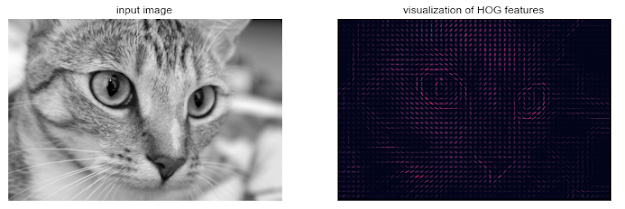## 2015年10月18日 星期日

### [ GroovyGN ] Multiple Assignments

Source From Here
Since Groovy 1.6 we can define and assign values to several variables at once. This is especially useful when a method returns multiple values and we want to assign them to separate variables.
1. // Assign and declare variables.
2. def (username, email) = ['mrhaki''email@host.com']
4. assert 'email@host.com' == email
5.
6. // We can assign later than the definition of the variables.
7. int housenr
8. String streetname
9. (streetname, housenr) = ['Old Street'42]
10. assert 42 == housenr
11. assert 'Old Street' == streetname
12.
13. // We can also use type declerations.
14. def (String streetName, int houseNumber) = ['Old Street'42]
15. assert 42 == houseNumber
16. assert 'Old Street' == streetName
17.
18. // Return value of method can be assigned to multiple variables.
19. def iAmHere() {
20.     [29.2009012.90391]
21. }
22. def (coordX, coordY) = iAmHere()
23. assert coordX == 29.20090
24. assert coordY == 12.90391
25.
26. // More values than variables: extra values are ignored.
27. def (a, b, c) = ['a''b''c''d']
28. assert 'a' == a
29. assert 'b' == b
30. assert 'c' == c
31.
32. // Less values than variables: variable is not set.
33. def (x, y, z) = 
34. assert 100 == x
35. assert 200 == y
36. assert !z
37.
38. // Useful for getting regular expressions matching groups.
39. def money  = '12 Euro'
40. def regexp = /(\d+) (\w+)/
41. def (exp, amount, currency) = (money =~ regexp)
42. assert '12' == amount
43. assert 'Euro' == currency
Supplement
The Simple Groovy datatypes - Working with regular expressions

### [Py DS] Ch5 - Machine Learning (Part14)

Application: A Face Detection Pipeline This chapter has explored a number of the central concepts and algorithms of machine learning. But mo...